# Introduction to Effective Field Theories in Elementary and Collider Physics

## Lecture: University of Vienna, WS 2010/2011

### Instructor: Prof. Dr. André Hoang

#### Prerequisits

• concepts of quantum field theory
• Standard Model of particle physics
• being comfortable computing Feynman diagrams

#### Aims

• Learning how to construct and apply effective field theories.
• Deepen and widen concepts of renormalization and renormalization group equations..
• See how the theory of quantum fields relates to physical processes.
• Have some fun, ... after all.

#### Textbooks

• Heavy Quark Physics, by Aneesh V. Manohar and Mark B. Wise (Amazon Info)
There is an official list of errors and misprints. Best book available on Heavy Quark Effective Theory (HQET).
• Dynamics of the Standard Model, by J. F. Donoghue, E. Golowich, B. R. Holstein, (Amazon Info)
Excellent text book demonstrating how Standard Model field theory is applied in particle phenomenology.
• Quantum Field Theory, by Mark Srednicki (Amazon Info)
Excellent modern field theory book telling you how things are done. Recommended !

#### Other useful References

• Many references are from recent scientific literature and given during the course.
• An Introduction to Quantum Field Theory, M. E. Peskin and D.V. Schroeder (Amazon Info).
There is an official list of errors and misprints.
• Renormalization, by J. Collins (Amazon Info)
• Quantum Field Theory in a Nutshell, A. Zee (Amazon Info)
• Gauge Theory of Elementary Particle Physics, T.-P. Cheng and L.F. Li (Amazon Info)
• Weak Interactions and Modern Particle Theory, by H. Georgi.
Out of print ... but available here for free! (You can make money with this book.)
Be aware of the convention this book uses for γ5.

#### Lecture

• Start: October 8, 2010
• Last lecture: January 28, 2011
• Friday, 10:15 - 11:45 (change of room: Erwin Schrödinger Hörsaal, Zi. 3500, physics building)
• Exercise class: Friday, 9:00-10:15. Erwin Schrödinger Hörsaal, Zi. 3500, 5. Stock. Start: October 21, 2010.

#### Office Hours and Infos

• Wednesday: 16:00 - 17:00. Send me email in advance.
• Mails to students: Oct. 8 , Oct. 14 , Oct. 19 , Oct. 23 , Oct. 30 , Nov. 3 , Nov. 8 , Nov. 16 , Nov. 21 , Nov. 30 , Dec. 7 , Dec. 14 , Jan. 9 , Jan. 14 , Jan. 24 , Feb. 1

#### Homework Problems

• Interesting and useful homework problems will be identified and mentioned in class weekly.
• Let me know if you are interested in having a tutorial.

#### Lecture Notes and Exercises

• Intro Lecture: Concepts of effective theories
• Lecture 1: Introduction to quantum chromo dynamics (QCD)
• Lecture 2: Basics of the QCD Lagrangian
• Lecture 3: FeynmanRules

• Exercises (October 29, 2010): (solutions by Pedro Ruiz-Femenia)
• (1) Rederive for yourself the various computations in deriving x-space Green functions in phi^4 theory as discussed in class and go to second order in lambda for the connected 2-point Green function. This gives you all relevant two-loop diagrams. Try to be efficient handling the computations !!! They can be short and efficient or tedious and time consuming.
• (2) Determine the momentum (Fourier) space 2-loop Green function as defined in class, i.e. using the convention of incoming external momenta. You should already use the contraint that the external momenta satisfy energy-momentum conservation, so not all external moments are independent. (For the mom-space connected Green function you can neglect the overal factor (2Pi)^4 times delta-function.)
• (3) Look at the results and set up rules how to get to the results without considering first the x-space computations. Are the symmetry fators modified when switching from position to momentum space?
• (4) Apply the rules of (3) to determine the Feynman diagrams of the connected 4-point function up to O(lambda^2) and determine the expressions for the diagrams im momentum space. If you have trouble determining the symmetry factors go through (1) to determine them explicitly. Then try to understand the rules for the symmetry factors you could not determine.

• Exercises (November 5, 2010): (solutions by Maximilian Stahlhofen)
• (1) Derive the Feynman rules (vertex factors and propagators) of QED in R_xi gauge. For the functional derivative w.r. to the electron fields (which are Grassmann quantities) you should use the convention that derivative of the barred electron fields always stands to the right, i.e. they act first. The solutions is already given in the notes.
• (2) Derive the Feynman rules (vertex factors and propagators) of QCD in R_xi and in axial gauge.

• Exercises (November 12, 2010): (solutions by Pedro Ruiz-Femenia)
• (1) Consider the generic generating functional W[α,α] for a single fermion theory with a generic fermion current source LI = ψ(x) V(x) ψ(x) which was discussed in the class. V(x) represents bosonic structures.
(a) Compute the connected 4-point Green function <0| ψ(x1) ψ(x2) ψ(x3) ψ(x4) |0> at tree level. The two diagrams you obtain have a relativ minus sign. Look back how this sign is generated and formulate the corresponding rule.
(b) Consider that V(x) represents an external source for the fermion current, i.e. you can consider it another bosonic particle that interacts with the fermions. Compute the Green function for a fermion loop generated from n such sources at x1, ..., xn.
• (2) The physics content of a field theory is unchanged by field redefinitions. Consider the free part of the Lagrangian of the φ4 field theory and make the field redefinition φ → φ+ λφ2. Work out the Feynman rules of the field-redefined theory and determine the scattering amplitude for φφ → φφ at tree level. Show that the amplitude is zero. This is because you are still having a free field theory in disguise.

• Lecture 4: Loops and Renormalization

• Exercises (November 19, 2010): (solutions by Maximilian Stahlhofen)
• (1) Computein d=4-2ε dimensions, where A is a real number. We assume that there are one time and d-1 spatial dimensions. You are supposed to do the computation in two different ways. Use Mathematica or tables when needed.
(a) First show that the total solid angle in n dimensions has the formand has the result.
(b) The first way is to use the Wick rotation in order to turn the d-Minkowski integral into an Euklidean integration.
(c) The second way avoids Wick rotation. Do the q0 integration by residues and then carry out the d-1 dimensional spatial integral afterwards.
• (2) Dimensional regularization regularizes UV as well as IR divergences. Sometimes both kinds appear in the same loop diagram, and it is necessary to distinguish them. Consider the scaleless integral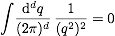. Convince yourself that it is UV and IR divergent for d=4. Idenfify the UV-divergence by making the massless propagators massive, q2+iε→ q2-m2+iε. Determine the form of the IR divergence for ε→ 0. Use Mathematica to expand the d-dimensional result for small ε.
• (3) Use Mathematica or tables to show the Feynman parameter relations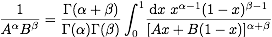and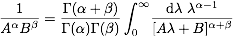. The first relation is used when A and B have the same mass dimensions and x is dimensionless. The second relation is used then A and B have different dimensions, and [λ]=[B]-[A].
• (4) Compute the integralin two ways. (The term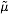is just a constant.)
(a) Use Wick rotation to turn the integral into a Euklidean integral such that you can then do the radial integration using Mathematica or tables.
(b) Use the first Feynman parameter relation to turn the integral into a form to use In from exercise (1). Use Mathematica or tables for the remaining Feynman parameter integral over x.

• Exercises (November 26, 2010): (solutions by Pedro Ruiz-Femenia)
• (1) Compute the contraction of gamma matrices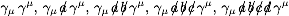and the tracesin 4 and d dimensions.
• (2) Derive the renormalized QED Lagrangian to one-loop order using the multiplicative renormalization relationsbetween bare and renormalized fields, mass and coupling. Use that to one-loop order one can expand the renormalization constants as.
• (3) Derive the Feynman rules of renormalized QED and write down the complete set of vertex functions (one-particle irreducible, amputated Green functions) to one-loop order. This amounts to the fermion and photon 2-point functions and the fermion-photon vertex. Write down the corresponding loop integrals and carry out gamma matrix contractions and traces in d dimensions.

• Exercises (December 3, 2010): (solutions by Maximilian Stahlhofen)
• (1) Compute the integralthat was used in class for the renormalization of the photon 2-point function.
• (2) Carry out the tensor reduction computations carried out in class for the one-loop integralsand.
• (3) Determine the one-loop MS counter terms for φ4-theory with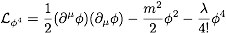. Proceed as was done in class for QED. In this exercise you need to apply most of what you have learned before. Do the simplest possible computations to get the results particularly for the 4-point functions. The results are relevant for the Higgs sector of the Standard Model.

• Exercises (December 10, 2010): (solutions by Pedro Ruiz Femenia)
• (1) Determine the MS leading logarithmic (one-loop) renormalization group equations of φ4-theory using the renormalization constant determined last week and compute their solutions as shown in class.
• (2) The renormalization group equations remain valid even if m2 is negative and φ get a vacuum expectation value. Set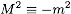and compute the vacuum expectation value v. Show that the scalar field has the mass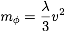. Now assume that φ4-theory is a model for the Standard Model Higgs. This model is actually quite accurate in many respects. We know that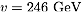and can use the MS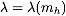in the Higgs mass expression. Determine the renormalization scale μ where the Higgs self coupling diverges and the theory for sure breaks down as a function of the Higgs mass. Which field theoretic and physical conclusions do you have to draw? Why - in this light - is LHC a no-loose experiment?
• (3) Take the ansatz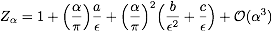for the QED coupling renormalization constant and determine the two-loop renormalization group equation pushing the computation we did in class to one higher order. Show that, if the renormalization group equation is finite for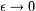, b is not independent but a function of a. Determine the function.

• Exercises (January 9, 2011): Asymptotic Expansion and Powercounting (solutions by Maximilian Stahlhofen)

Consider the following one-dimensional integral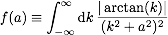. You can think of this integral as being a simplified version of a loop-Feynman diagram where the denominator corresponds to a propagator structure. The concepts discussed in this exercise apply to usual Feynman diagrams as well. It is your task to compute the expansion for small a<<1. Naive expansion in a before integration does not work because of an IR singularity. You might want to compute the integral exactly and then expand the result for small a, but this is very difficult. Instead use the two methods below. Use Mathematica for the computations so same some work.
• (1) Cutoff Method:
In the limit a « 1 the integral is governed by the two regions k<<1 ("hard") and k &sim a << 1 ("soft"). That's the reason why naive expansion does not work. Separate the soft and the hard regions by introducing a cutoff &Lambda with a << &Lambda << 1 which splits the integral into two parts, |k|< &Lambda and |k|> &Lambda. Carry out the Taylor expansions that now become possible in the two regions and do the integrations. Expand the individual results of the integration using that a<< &Lambda << 1 and add back together the results. In this way determine the expansion of f(a) neglecting term at order a2 or higher. Check your result numerically with Mathematica.
Since &Lambda has been introduced by hand the result should be independent of it at any order in the a expansions. Which problem emerges?
• (2) Dimensional Regularization:
You can use dim reg to do a similar computation. While the cutoff method is probably quite intuitive and easy to understand for you, using dim reg involves a number of subtle issues you have to get used to, but it is more powerful in practice. So, first continue the integral to D=1-2&epsilon,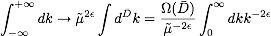. where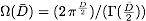is the D-dimensional angular integral and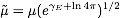.
Expand the integrand for the soft regime (a,k<<1) as described in (1), integrate the terms in D dimensions and expand for &epsilon &rarr 0. Remember what you have learned in class about doing integrations in dim reg. Have a look at the terms you obtained in the expansion for small k before integration and observe the order in a they contribute. Establish so-called power counting rules for the soft regime that tell you (before integration!) to which order each term contributes. Determine all terms up to (including) order a.
Expand the integrand for the hard regime (k &sim 1) as described (1), carry out the integral in D dimensions and expand for &epsilon &rarr 0. Establish the power counting rules in the hard regime and determine all terms up to (including) order a.
Now add the contributions you got from the expansions in the soft and the hard regime. The result is the expansion of f(a) for small a and should agree with your result from (1). (If you are motivated, compute also the order a2 terms.) Think about how this could have all worked out. Which way of computing the expansion do you find more attractive?

• Lecture 5: Decoupling, Integration out Heavy Particles and Basics of Constructing Effektive Theories

• Exercises (January 14, 2011): Matching with Massive Electrons (solutions by Pedro Ruiz-Femenia)
Consider the standard QED describing the dynamics of electrons and photons. As discussed in class, for photon momenta qμ much smaller than me one can integrate out the electrons and work with a low-energy effective theory containing only the photons. This effective theory is called the Euler-Heisenberg theory.
• (a) Determine the one-loop photon vacuum polarization diagram with dimensional regularization as discussed in class in the MS renormalization scheme and expand it for small q2/me2. Explain why the first term in the expansion motivates matching onto the effective theory at the scale μ of order me rather than, let's say, at μ of 100 TeV. The usual convention is to match at μ=me.
• (b) Take the dimension-6 photonic operator in the effective theory discussed in class and show that its Feynman rule can reproduce the momentum-dependence of the second term in the expansion of the one-loop vacuum polarization function. Fix the Wilson coefficient c1(μ=me) of the dimension-6 operator such that it reproduces the second term exactly. Determine the Wilson coefficients of the effective theory up to dimension-6 (c0, c1) for matching at a scale μ≠ me.
• (c) You need to check whether what you did is unambiguous by showing that there is no other photonic dimension-6 operator that is gauge-invariant and might do the same job. Write down a few other possibilities and use integration by parts and the equation of motion in the effective theory ∂μFμν=0 to show that other possibilities either vanish or reduce to the one you already used in part (b).
• (d) Look up how the electron and photon fields transform under C, P and T and show that QED is C-,P- and T-invariant. Show why these symmetries forbid dimension-6 operators with three field strengths from ever appearing. (This feature is also known as Furry's Theorem''.)
• (e) At dimension-8, operators are generated which describe light-by-light scattering (γγ→γγ). Write down QED one-loop diagrams that can match onto these operators and determine the power of α contained in their Wilson coefficients. Use this information and simple dimensional analysis in the effective theory (e.g. take all numbers that arise of order one, but keep momenta, masses and couplings) to obtain a numerical estimate for the cross section γγ→γγ for 10 keV photons. Does the reaction happen at a large rate? Compare to QED cross sections you might have computed before in other lectures.

• Exercises (January 24, 2011): Gauge Coupling Unification in a (SUSY) SU(5) GUT (solutions, dynamic Mathematica notebook showing the effects of SUSY particles (you need to evaluate all definitions to view the dynamic plot), by Maximilian Stahlhofen)
We discussed SU(5) gauge coupling unification in class. Assuming a SU(5) gauge symmetry exists at very high energy scales with a gauge coupling gGUT one can argue that the symmetry is broken (e.g. by some Higgs mechanism) down to SU(3)×SU(2)×U(1) at some scale μ=MGUT. If MGUT is very much larger than the electroweak scale, one should integrate out all the heavy fields and switch to an effective theory that has the symmetries SU(3)×SU(2)×U(1). (Since this is all a model, one can just assume that all the heavy particles have similar mass of order MGUT.) When doing the matching computations at the GUT scale one finds that the breaking patten requires that the gauge couplings for the unbroken symmetries, gs, g2, g1 are functions of the original gauge couplings,
gs=gGUT,   g2=gGUT,   g1=√(3/5) gGUT.

(where s=SU(3),2=SU(2),1=U(1)). One can test whether this unification idea is consistent with low energy data using the measured results for the $\overline{\rm MS}$ couplings obtained from experiments at LEP (μ=MZ=91.2 GeV),
sin2W)(MZ)=0.232,    α(MZ)=(128.9)-1,    &alphas(MZ)=0.118± 0.003.

The error in the electromagnetic coupling and the Weinberg angle is at the level of 0.1%.
• (a) Assume that the effective low energy theory below MGUT is the Standard Model. Determine the values for the MS $MS} couplings αs=(gs)2/(4π), α2=(g2)2/(4π) and α1=5(g1)2/(12π) at the scale MZ. The LL RGE's for these couplings have the formwith b1=-2/3nf-1/10nh, b2=22/3-2/3nf-1/6nh, b3=11-2/3nf, where nf is the number of quarks and nh the number of Higgs doublets. (You can treat the top quark as a light quark in this context. Think about why this is still a valid approximation in this case.) Compute the LL solution for the running couplings above MZ taking the values at MZ as an input. Is the SU(5) unification scenario consistent with low energy data? • (b) Assume that the effective theory below MGUT is not the Standard Model, but the minimal supersymmetric Standard Model (MSSM). Since no supersymmetric (SUSY) partner of any Standard Model has ever been seen, this scenario is only possible if all SUSY partners are much heavier than any of the Standard Model particles. Let us assume that all SUSY partners have similar masses of order$MSUSY. So for scales below \$MSUSY one can integrate out the SUSY partners finally arriving at the Standard Model as the effective theory for scales below MSUSY. The matching conditions for the gauge couplings at μ=MSUSY are just as discussed in class. The MSSM anomalous dimensions have the form b1=-nf-3/10nh, b2=6-nf-1/2nh, b3=9-nf, where nf is the number of quarks (in the Standard Model) and nh the number of Higgs doublets. (In the MSSM one has two Higgs doublets!) Compute the LL solution for the running couplings above MSUSY taking the values at MZ as an input. (Note that this can be done in very compact form.) Can you find scales MSUSY and MGUT such that unification is realized at the scale MGUT? Note that you should account for the fact that the low energy data for the couplings have experimental uncertainties. Is the SUSY SU(5) unification scenario consistent with low energy data? Which scales for MSUSY are the ones most favored by the analysis?
• (c) Think about the conditions that needed to be satisfied to make the LL analysis carried out above valid. What does the analysis tell you? Discuss the physical implications.

#### Maximal Syllabus (we will not cover all of the items)

• Introductory Part
• Review of the Standard Model
• Standard Model as part of an effective theory.
• Renormalization and Loops, Part I
• Decoupling,
• EFT for QED with massive fermions,
• EFT for the Standard Model for heavy top, W, Z
• Unification of gauge couplings
• Renormalization and Loops, Part II (mixing)
• General Aspects of QCD
• Behavior of High-Order Perturbation Theory
• Power Corrections
• Renormalons
• Heavy-Quark-Effective Theory
• Symmetries, Action, Power Counting
• Form Factors, CKM Matrix Elements
• Non-perturbative Corrections
• Inclusive, Semileptonic Decays
• Non-relativistic QCD
• Degrees of Freedom, Action, Velocity Power Counting
• Derivation of the Nonrelativistic Schroedinger Equation
• Lamb-Shift
• Chiral Perturbation Theory
• Chiral Symmetry, Anomalies, Power Counting
• Electromagnetic Processes
• Pion, Kaon Processes
• QCD Factorization, Soft Collinear Effective Theory
• Collinear Fields and Operators, Symmetries, Power Counting
• Factorization
• Deep Inelastic Scattering
• B Decays into light Hadrons
• Aspects of Jet Physics